# Formulas for the Determination of Yarn Count

The system by which we determine the yarn number is called count. Count is the number of yarn denoting the sophistication or obesity of yarn. So by linear density or the yarn count, we can know how fine or course the yarn is. It indicates the mass per unit length or length per unit mass of yarn.

The length of the sample X Weight unit
-         Indirect count system = ………………………………………………

The weight of the sample X Length unit

From the above formula, the count in different indirect systems is as follows –
Sample yarn length (yds) X 1 lb
1.     English cotton count (Ne) = ………………………………………………
Sample yarn weight (lb) X 840 yds
Sample yarn length (m) X 1 kg
2.     Metric count (Nm) = ………………………………………………
Sample yarn weight (kg) X 1000 m
Sample length (m) X 0.5 kg
3.     French or Continental count (Nf) = …………………………………………
Sample weight (kg) X 1000 m
Sample length (yds) X 1 lb
4.     Worsted count (w) = ………………………………………………
Sample weight (lb) X Weight unit

Sample yarn weight X length unit
-         Direct count system = ……………………………………………
Sample yarn length X Weight unit

From the above formula, the count in different direct systems is as follows –
Sample yarn weight (gm) X 1000 m
1.     Tex count = ……………………………………………………
Sample yarn length (m) X 1 gm
Sample weight (gm) X 9000 m
2.     Denier count = ………………………………………………
Sample length (m) X 1 gm
Sample weight (lb) X 14400 yds
3.     Linen/Hemp/Jute count = …………………………………………………
Sample length (yds) X 1 lb

### Conversion of count from one count to another

1.     Denier to cotton:
English cotton count = 5315/Yarn count in denier system
2.     Denier to Tex:
Tex count = Count in denier system/9
3.     English to Tex:
Tex count = 590.5/English count
4.     English to denier:
Denier count = 5315/English count
5.     Cotton to metric:
Metric count = English cotton count X 1693
6.     Tex to denier:
Denier = Tex X 9

### Resultant count of yarn (For same count of yarn)

1.     Resultant count of indirect system = Count of single yarn/No. of doubling or ply
2.     Resultant count of direct system = Count of single yarn X No. of doubling or ply

### Resultant count of yarn (For different count of yarn)

1.     Resultant count of indirect system = 1/Sum of the weight of folded yarn’s length unit
2.     Resultant count of direct system = Sum of the weight of folded yarn’s length unit

### Mathematical problems and solution of yarn count

1.     Find out the cotton count if weight of 8400 yds yarn is 1 lb.
Solution:
We know,
Length (yds)                 1 lb
English cotton count =…………………….. X …………………
840 (yds)                      Weight (lb)
8400 yds                            1 lb
= ……………………… X …………………… = 10 S (ANS).
840 yds                              1 lb

2.     If 120 yds cotton yarn having 25 grain weight then what will be the count?
Solution:
Yarn length (yds)                    1 lb
English cotton count = ……………………………. X ……………………….
840 yds                               Yarn weight (lb)
= 120/840 X 1/(25/7000) = 120/840 X 7000/25
= 40S (ANS).

3.     If 1000m Nylon yarn having 1.67gm weight then what will be the Denier?
Solution:
We know,
Yarn wt. (lb)                           14400 yds
Jute count = ……………………….. X ………………………
1 lb                                   Yarn length (yds)
3/16                    14400 yds
= ……………… X ………………….
1 lb                       100 yds
= 3/16 X 14400/100 = 27 lb/Spindle (ANS).

4.     Find out the metric count if weight of the 400m yarn is 40gm.
Solution:
We know,
Length (m)                     1 kg
Metric count = …………………….. X …………………
1000 m                          Weight (m)
= 400m/1000m X 1kg/(4kg/1000) = 10S (ANS).

5.     Find out the worsted count if the weight of 80 yds is 50 grain.
Solution:
We know that,
Length (yds)                  1 lb
Worsted count = ……………………… X …………………
560 yds                          Weight (lb)
80 yds                              1 lb
= …………………… X …………………….
560 yds                            50/7000 lb
= 20S (ANS).

6.     A trading corporation provides you a 24.3 Ne yarn, Find out the Tex and denier of that yarn.
Solution:
Given,
Wk = 1 lb = 453.6 gm
Wr = 1 gm
Ik = 840 yds = 840 X 0.9144 m
Ir = 1000 m
We know,
1                     Wk/Wr
Nr = ………… X ………………
Nk                     Ik/Ir
1                                     453.6/1
Tex = ……………. X …………………………………..
24.3                         (840 X 0.9144)/1000
= 24.3 Tex (ANS).
Again,
We know denier = 9 X Tex
= 9 X 24.3 Tex = 218.7 denier (ANS).
Previous Post
Next Post

#### Md. Abu Sayed

He is a Textile Engineer and like writing on Apparel

#### 13 comments:

1.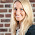Dear Abu, thanks for this interesting blog. Will use whenever I need specific information, cheers from Germany, Heike

1.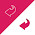You are most welcome Dear. Thanks for your sweet comment.

2.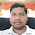Count =30 & 40
Resultant count =?
Plz describe it

3.For 30 count, wt. of 1 hank yarn = 1/30 lbs
and for 40 count, wt of 1 hank yarn = 1/40 lbs
So, the resultant count = {1/ (1/30+ 1/40)} = 17.14 S

4.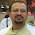what is formula to convert hank into kg

5.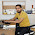840 yard=770 m=1 Lea=120 yard=0.45kg
1 Hank = 120 yard
Then we will do calculate number of hank *120 yard

6.1 Hank = 120 yard
Total number of hank multiple from 120 yard
840 yard = 770 m= 120 Yard= 110 m
110m = 0.45 kg

7.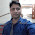nice information

8.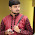A good consolidate information. nice to know.
Can you please share the process how to find out the yarn count and type of 280GSM 80/20 CVC? both for blended and polyester in the binder.

9.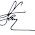count 15 Tex, 20 Tex and 25 Tex ,,calculate the resultant count..
please solve it....

10.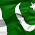please share how to calculate the length of Nylon 20/S?

11.what is 1693 in yarn weight calculation, why we have to use this ?

12.It's very useful. The Yarn Count Test Machine is an automated instrument that solves the problem of how to measure yarn count. The Yarn Counting Machine uses the international yarn count formula and is automatically tested by the yarn count calculator. It is simple and practical. If you want to know more, welcome to visit on https://www.testextextile.com/product/yarn-count-tester-ty361/